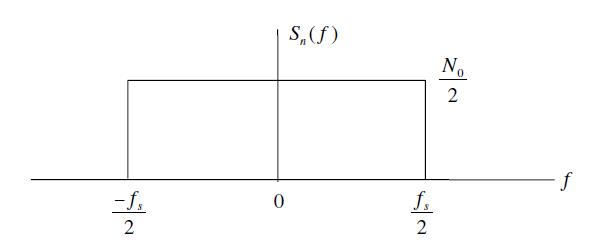# A Comparison of FFT, MUSIC and ESPRIT Methods of Frequency Estimation

As discussed in previous posts it is frequently required in communications and signal processing to estimate the frequency of a signal embedded in noise and interference. The problem becomes more complicated when the number of observations (samples) is quite limited. Typically, the resolution in the frequency domain is inversely proportional to the window size in the time domain. Sometimes the signal is composed of multiple sinusoids where the frequency of each needs to be estimated separately. Simple techniques such as Zero Crossing Estimator fail in such a scenario.  Even some advanced techniques such as MATLAB function “pwelch” fail to distinguish closely spaced sinusoids.

Continue reading A Comparison of FFT, MUSIC and ESPRIT Methods of Frequency Estimation

# MSK – A Continuous Phase Modulation (CPM)

## Some Background on MSK

`I – In the previous post we presented the mathematical model and code for BER calculation of a popular modulation scheme called MSK. However in the code we shared, we only considered one sample per symbol, which makes MSK look like BPSK. While BPSK symbols fall on the real axis, MSK symbols alternate between real and imaginary axes, progressing by π/2 phase during each symbol period. MSK signal thus has memory and this can help in demodulation using advanced techniques such as Viterbi Algorithm. `
Continue reading MSK – A Continuous Phase Modulation (CPM)

# Noise Calibration in Simulation of Communication Systems

We have been using a wireless signal model in our simulations without going into the details of noise calibration for simulation. In this article we discuss this. Lets assume the received signal is given as

r(t)=s(t)+n(t)

where r(t) is the received signal s(t) is the transmitted signal and n(t) is the Additive White Gaussian Noise (AWGN). Channel fading is ignored at the moment. Signal to noise ratio for simulation of digital communication systems is given as

ρ=Eb/No (1)

Where Eb is the energy per bit and No is the noise Power Spectral Density (PSD). We also know that for the case of Additive White Gaussian Noise the noise power is given as [Tranter]

σ^2=(No/2)*fs

No=2*(σ^2)/fsWhere σ is the standard deviation of noise and fs is the sampling frequency.  Substituting in equation 1 we get

ρ=Eb/No=Eb/(2*σ^2/fs )

Eb/No=(Eb*fs)/(2*σ^2)

σ^2=(Eb*fs)/(2*Eb/No )

σ=√((Eb*fs)/(2*Eb/No ))

If the energy per bit and the sampling frequency is set to 1 the above equation reduces to

σ=√(1/(2*Eb/No ))

The simulation software can thus calculate the noise standard deviation (or variance) for each value of Eb/No in the simulation cycle. The following piece of MATLAB code generates AWGN with the required power and adds it to the transmitted signal.

```s=sign(rand-0.5);      % Generate a symbol
sigma=1/sqrt(2*EbNo);  % Calculate noise standard deviation
n=sigma*randn;         % Generate AWGN with the required std dev
r=s+n;                 % Add noise to the signal```

How can we assume that energy per bit and sampling frequency is equal to one and are we breaking some discrete time signal processing rule here. This will be discussed in a later post.